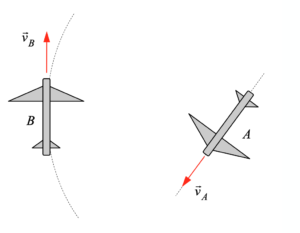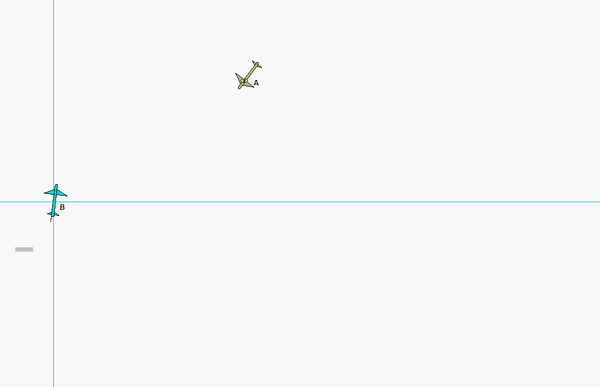# Moving reference frames - aircraft problemAircraft A and B are flying on the same horizontal plane, each with a constant speed. Aircraft A is traveling on a straight path, whereas the path of B is circular. We are interested in understanding how the motion of one aircraft is viewed by a passenger on the other aircraft.

Stationary observer
Shown below is an animation of the motion of A and B as seen by a stationary observer. The paths of A and B are straight and circular, as expected.View of B as seen by an observer on A
Shown below is an animation of the motion of aircraft B as seen by a passenger on aircraft A. The path of B is no longer circular, as expected. Can you see this in the relative motion equation for (vB/A)rel ?View of A as seen by an observer on B
Shown below is an animation of the motion of aircraft A as seen by a passenger on aircraft B. The path of A is no longer straight, as expected. Can you see this in the relative motion equation for (vA/B)rel ?NOTE:
Recall from before that when the observer is not rotating, then (vA/B)rel  = -(vB/A)rel . In the case, an observer on B IS rotating, and as we can see in these animations, these two observed velocities differ by much more than a sign.